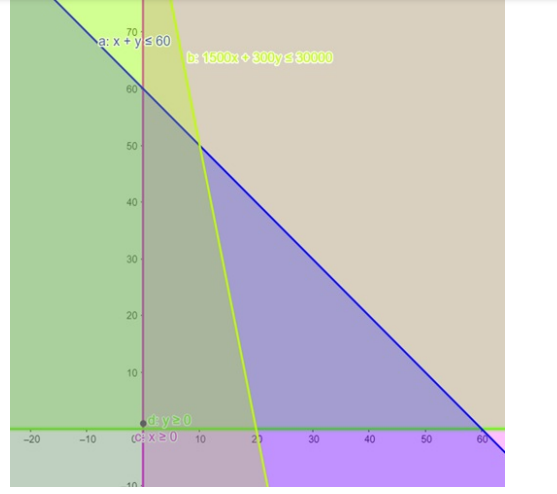# A furniture dealer deals in only two items : tables and chairs.

Question:

A furniture dealer deals in only two items : tables and chairs. He has 30000 to invest and a space to store at most 60 pieces. A table costs him 1500 and a chair 300 . Formulate the data in the form of inequations and draw a graph representing the solution of these inequation.

Solution:

Let the number of tables and chairs be $x$ and $y$ respectively.

Therefore $x \geq 0, y \geq 0$

Now the maximum number of pieces he can store $=60$.

Therefore, $x+y \leq 60 \ldots \ldots$ (1)

Also it is given that maximum amount he can invest $=30000$

Therefore, $1500 x+300 y \leq 30000 \ldots \ldots$ (2)Therefore, the shaded protion (i.e. A) together with its boundary represents the solution set of the given inequation.

No. of tables $=x=10$

No. of chair $=y=50$